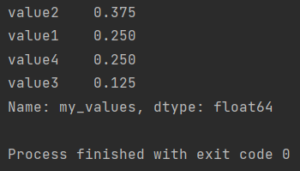# How to count specific value in column

Here is how to count specific value in column in Pandas.To count the number of values in a column use the value_counts function in Pandas.

## How to count specific value in column

```import pandas as pd

df1 = pd.DataFrame({"my_column": ["string1", "string2", "string3", "string4",
"string5", "string6", "string7", "string8"],
"my_values": ['value1', 'value2', 'value1', 'value3',
'value4', 'value2', 'value4', 'value2']})

print(df1)
print(df1['my_values'].value_counts())

```

The output:

```  my_column my_values
0   string1    value1
1   string2    value2
2   string3    value1
3   string4    value3
4   string5    value4
5   string6    value2
6   string7    value4
7   string8    value2

value2    0.375
value1    0.250
value4    0.250
value3    0.125
Name: my_values, dtype: float64

Process finished with exit code 0

```

Instead of counting a number of values, you can also display the ratio of the values.

## How to get proportion of values

To get the proportion use an additional parameter of value_counts method. Normalize = True gives you the proportion instead of the number of occurrences of the value.

```import pandas as pd

df1 = pd.DataFrame({"my_column": ["string1", "string2", "string3", "string4",
"string5", "string6", "string7", "string8"],
"my_values": ['value1', 'value2', 'value1', 'value3',
'value4', 'value2', 'value4', 'value2']})

print(df1['my_values'].value_counts(normalize=True))

```
```value2    0.375
value1    0.250
value4    0.250
value3    0.125
Name: my_values, dtype: float64

Process finished with exit code 0
```

Link to the documentation of value_counts method.

## How to get summary of values

Instead of the number of values, you can also get a summary by using the describe function. In addition to the number of values, you will also get other statistical values.

`import pandas as pd`

df1 = pd.DataFrame({“my_column”: [“string1”, “string2”, “string3”, “string4”,
“string5”, “string6”, “string7”, “string8”],
“my_values”: [‘value1’, ‘value2’, ‘value1’, ‘value3’,
‘value4’, ‘value2’, ‘value4’, ‘value2’]})

print(df1[‘my_values’].describe())

Link to the documentation for the describe function.